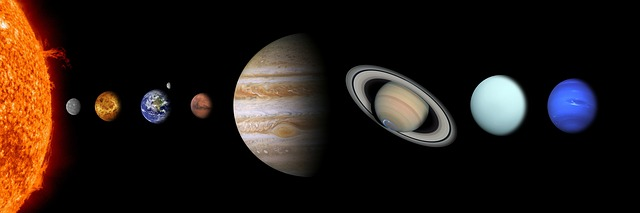# Solution to problems – class 9 – Set 1 Q36

Last updated on June 17th, 2019 at 02:48 pm

## Problem Statement

The mass of sun is 2 × 10^30 kg and the mass of earth is 6 × 10^24 kg. If the average distance between the sun and the earth be 1.5 × 10^8 km, calculate the force of gravitation between them.

## Solution

M1 = mass of the sun = 2 × 1030 kg
M2 = mass of the earth = 6 x 1024 kg

Distance between the sun and the earth = r = 1.5 × 108 km = 1.5 × 1011 m

Gravitational force between the sun and the earth = F

F = (G M1 M2) / r2
= (6.7 x 10 -11) (2 × 1030  x 6 x 1024) / (1.5 × 1011) 2
= 3.57 × 1022 N

Question Set – Physics Problems for grade 9

See also  Solution of Physics Numericals for class 9 (set 1 Q11)
Scroll to top
error: physicsTeacher.in